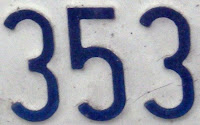## Monday, February 15, 2010

### 353

353 is a prime number.

353 has a representation as a sum of two squares: 353 = 82 + 172.

353 is the hypotenuse of a primitive Pythagorean triple: 3532 = 2252 + 2722.

353 is the smallest multidigit palindromic prime whose digits are all prime numbers.

666 is the sum of two consecutive palindromic primes: 313 + 353 = 666.

353 is the smallest prime for which its fourth power can be written as the sum of four integers to the fourth power: 3534 = 30 4 + 120 4 + 272 4 + 315 4.353 Ruperto-Carola is a small asteroid discovered by Max Wolf in 1893 in Heidelberg.